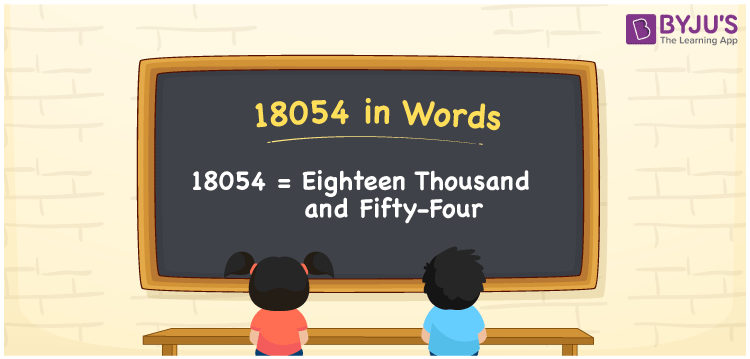# 18054 in Words

18054 in words is written as Eighteen thousand fifty-four. In both the International System of Numerals and the Indian System of Numerals, 18054 is written as Eighteen thousand fifty-four. The number 18054 is a Cardinal Number as it denotes some quantity. For example, “the laptop costs 18054 rupees”.

 18054 in Words Eighteen thousand fifty-four Eighteen thousand fifty-four in Number 18054

## 18054 in English Words

18054 in English words is read as “Eighteen thousand fifty-four”.## How to Write 18054 in Words?

To write 18054 in words, we shall use the place value chart. In the place value chart, put 1 in the ten thousands, 8 in the thousands, 0 in the hundreds, 5 in the tens and 4 in the ones. Let us make a place value chart to write the number 18054 in words.

 Ten Thousands Thousands Hundreds Tens Ones 1 8 0 5 4

Thus, we can write the expanded form as

1 × Ten Thousand + 8 × Thousand + 0 × Hundred + 5 × Ten + 4 × One

= 1 × 10000 + 8 × 1000 + 0 × 100 + 5 × 10 + 4 × 1

= 10000 + 8000 + 000 + 50 + 4

= 18054

= Eighteen thousand fifty-four.

18054 is a natural number, the successor of 18053 and the predecessor of 18055.

18054 in words – Eighteen thousand fifty-four

• Is 18054 an odd number? – No
• Is 18054 an even number? – Yes
• Is 18054 a perfect square number? – No
• Is 18054 a perfect cube number? – No
• Is 18054 a prime number? – No
• Is 18054 a composite number? – Yes

## Frequently Asked Questions on 18054 in Words

Q1

### How to write 18054 in words?

18054 in words is written as Eighteen thousand fifty-four.
Q2

### How to write 18054 in the International and Indian System of Numerals?

In both, the system of numerals, 18054 in words, is written as Eighteen thousand fifty-four.
Q3

### How to write 18054 in a place value chart?

In the place value chart, write 1 in the ten thousands, 8 in the thousands, 0 in the hundreds, 5 in the tens and 4 in the ones, respectively.